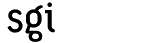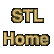# partitionCategory: algorithms Component type: function

### Prototype

```template <class ForwardIterator, class Predicate>
ForwardIterator partition(ForwardIterator first,
ForwardIterator last, Predicate pred)
```

### Description

Partition reorders the elements in the range [first, last) based on the function object pred, such that the elements that satisfy pred precede the elements that fail to satisfy it. The postcondition is that, for some iterator middle in the range [first, last), pred(*i) is true for every iterator i in the range [first, middle) and false for every iterator i in the range [middle, last).  The return value of partition is middle.

### Definition

Defined in the standard header algorithm, and in the nonstandard backward-compatibility header algo.h.

### Requirements on types

• ForwardIterator is a model of Forward Iterator.
• Predicate is a model of Predicate.
• ForwardIterator's value type is convertible to Predicate's argument type.

### Preconditions

• [first, last) is a valid range.

### Complexity

Linear. Exactly last - first applications of pred, and at most (last - first)/2 swaps.

### Example

Reorder a sequence so that even numbers precede odd numbers.
```int A[] = {1, 2, 3, 4, 5, 6, 7, 8, 9, 10};
const int N = sizeof(A)/sizeof(int);
partition(A, A + N,
compose1(bind2nd(equal_to<int>(), 0),
bind2nd(modulus<int>(), 2)));
copy(A, A + N, ostream_iterator<int>(cout, " "));
// The output is "10 2 8 4 6 5 7 3 9 1". 
```

### Notes

 The relative order of elements in these two blocks is not necessarily the same as it was in the original sequence. A different algorithm, stable_partition, does guarantee to preserve the relative order.